# Speed, time, distance ℹ️ rule and formulas for finding physical quantities, tasks and examples of calculations for grade 4 students, table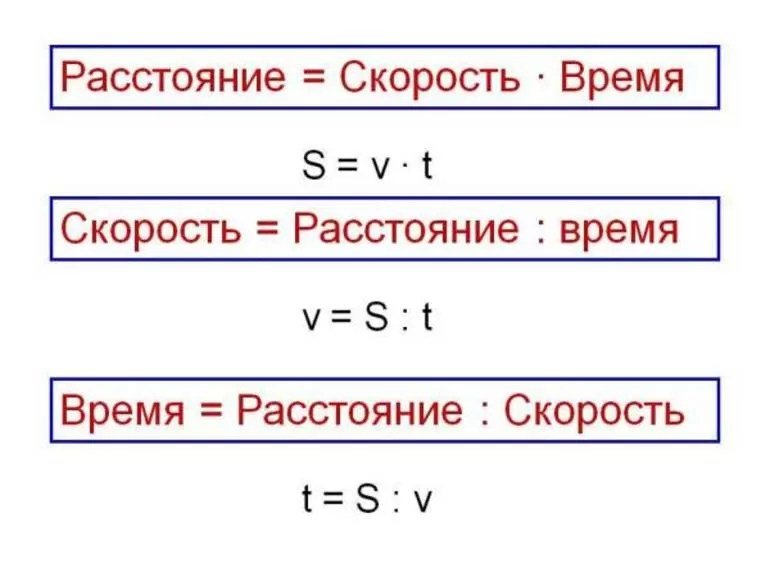## Time concept

There is a characteristic that one has to face every day, regardless of age, social status, various abilities and skills. With its help, the future, past and present are determined. Basically, it is a marker that defines an event. They call it time. Considering the movement, they always take into account this characteristic, as well as its progression.

Time is part of the spatial coordinate. But if you can move in different directions relative to other axes, relative to it, movement is determined only forward or backward. An integral part associated with time is space, thanks to which it is possible to understand the essence of the parameter.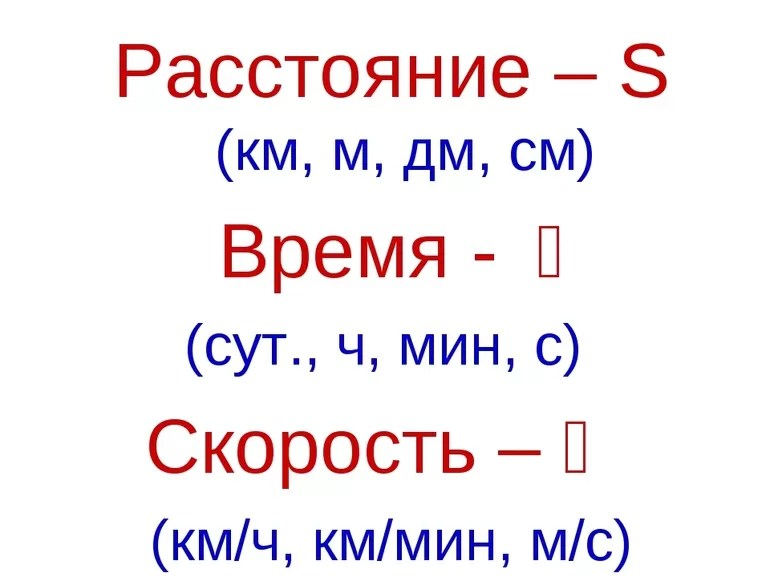The study of the characteristics was carried out by philosophers and scientists in different periods of the existence of mankind. It is impossible to see and hear time, unlike tangible space, which can be observed immediately and everywhere. Moreover, you can move in it.

Discussions on how to perceive time correctly are still ongoing. Plato believed that it is nothing more than movement. Aristotle assumed that time is a quantitative measurement of movement. It was added to the classical geometry of Euclid, acting on a limited number of dimensions. As a result, four-dimensional space began to be considered.

Today, there are still no answers to the following questions about time:• because of what it flows;
• why it is defined in only one direction;
• whether the parameter is one-dimensional, as many scientists believe;
• whether it is possible to detect quanta of the characteristic.

In classical physics, a special space-time coordinate is used to determine temporal change. It is customary to designate future events with a plus sign, and past events with a minus. The unit of measurement of time is associated with the rotation of the planet around its axis and the Sun. This choice was made conditionally and tied to the convenience of human life.

In the International System of Units, it is customary to take the interval equal to 9 192 631 770 periods of radiation of the cesium-133 atom at rest at zero degrees Kelvin per second. The parameter is denoted by the Latin letter t. Thus, time is a physical quantity associated with the movement of a body relative to the selected coordinate system.

## Distance and speed

The position of each physical point can be described using coordinate axes. In other words, a system that remains unchanged in relation to the investigated body. A change in position relative to another object can be represented by the distance traveled. In fact, it is a path for which the beginning and the end are known. From a physical point of view, distance is a quantity that is the dimension of length and expressed in its units.

In mathematics, the measure of the distance traveled is closely related to the metric space, that is, the position where the pair (x, d) exists, defined in the Cartesian product. Respectively, if the coordinate is taken as x, y, we can say the following:• the beginning of the path and its end are designated by points with coordinates d (x, y) and p (x, y);
• the distance traveled can be determined by subtracting the initial coordinates from the final coordinates;
• the change in position will be zero when d = p.

In physics, distance is measured in units of length. In accordance with the SI, meter is taken as the dimension. Distance is a measure of the distance traveled, that is, length. If you just need to determine the change in position without taking into account when and how it happened, use the coordinate axes. But when finding the distance traveled in time, one more quantity must be taken into account in the formula for the distance - speed.

This characteristic is designated by the symbol V. It characterizes the speed of movement in the selected frame of reference. By definition, speed is equal to the derivative of the point's radius vector with respect to time. In other words, it is a value specified by a position in space relative to an unchanged coordinate, which is most often taken as the origin.

The same distance can be covered in different times. For example, to walk 7 kilometers, a person will need to spend about one hour, but by car, this path can be covered in 10 minutes, or even less. These are the differences that depend on the speed of movement.

But in reality, not everything is so simple. The speed does not have to be the same all the way. At certain intervals, it can increase or decrease, therefore, in mathematics, its value is understood as the average value. The body is considered to move evenly over a specified distance.

## General formula

Speed, time, distance are 3 fundamental quantities related to each other. When examining one characteristic, it is imperative to consider the other two. In fact, speed is a physical quantity that determines how long a physical body will travel per unit of time. For example, a value of 120 km / h indicates that the object will be able to cover 120 kilometers in one hour. In mathematical form, the relationship between the three characteristics can be written as the following formula:

S = V * t, where:• S is the distance traveled by the object;
• V is the average speed of the body;
• t is the time taken to overcome the path.

Knowing this equality and any 2 parameters, you can calculate the third one, so for time it will look like t = S / V, and speed V = S / t. You can check the correctness of the formula for the speed of time and distance by analyzing the dimensions. If we substitute units of measurement into the expression, then after the reduction, a value corresponding to the determined value should be obtained. S = V * t = (m / s) * s = m (meter). What was required to receive. Similarly, you can check the 2 remaining formulas: t = s / v = m / (m / s) = m * s / m = s (second) and V = S / t = m / s (meter per second).

Indeed, let there be a physical body located in some place. After a while, no matter for what reasons, it moved to another point, while not leaving the established space. If the body is represented in the Cartesian plane, and the origin is taken as the coordinate (0, 0), after a while the object will change its position, which is determined by the value (x1, y2). In two-dimensional space, this change can be described as a transition from point A to B.Means, for the body to reach the second coordinate, it needs to spend time ... In this case, the path traveled will be in direct proportion to it. Distance and time should be linked by the third quantity, which is speed. That is, a parameter that determines how long it takes for a body to overcome a certain length.

As you can see, the expression connecting the 3 quantities is quite simple. But it does not take into account that the speed can be unstable, therefore, if the object travels its path unevenly, the average value is substituted into the expression. It is found as the sum of all individual speeds in uneven sections: Vav = ΔS / Δt.

## Solving problems

You only need to know one formula to be able to solve simple middle school math problems related to movement. In this case, it is necessary to pay close attention to the dimension. All calculations are performed in SI. Here are some of the typical assignments used to teach students in the fourth grade of high school: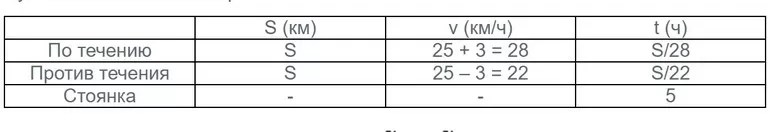1. A convoy of trucks drove out of settlement A to point B. A passenger car went to meet them. The speed of the carriers is 80 km / h, and the speed of the passenger car is 60 km / h. They met at point C an hour and a half later. Determine the distance between A and B. The solution to this problem will consist of several steps. On the first one you can find the path that the column traveled: 80 * 1.2 = 96 km. On the second, calculate the distance traveled by the second: 60 * 1.2 = 72 km. Hence, the total path will be equal to the sum: АС + СБ = 72 + 96 = 168 km.
2. The ship, whose speed in still waters is 30 km / h, goes with the flow, and then returns. The speed of the river is three kilometers per hour, with an intermediate stop taking 5 hours. The journey from start to return takes 30 hours. Find how many kilometers the entire flight is. To solve the problem, it is convenient to create a table. In the columns, you need to write down the distance, speed and time, and in the rows, the calculated data for events such as parking, travel upstream and downstream. Given the condition, the working formula will take the form: (S / 28) + (S / 22) + 5 = 30. The expression can be simplified. As a result, you should get: 25 * S / 308 = 25 → S = 308. Since the path of the ship consisted of two equal distances, the required distance will be: P = 2 * S = 308 * 2 = 616 km.
3. The train passes the bridge in 45 seconds. The length of the crossing is 450 meters. At the same time, the switchman, looking straight ahead, sees a passing train for only 15 seconds. Find the length of the train and the speed of its movement. If we assume that the train is moving at a speed V, then its length will be D = 15 * V. Since the train travels a distance of 45 * V = 450 + 15 * V in 45 seconds, it is easy to determine the speed from equality: V = 45 * V - 15 * V = 450 → V = 450/3 0 = 15 m / s. Therefore, the length of the train: D = 15 * 15 = 225 m.

All movement tasks can be divided into several types: moving towards, moving in pursuit, finding parameters with respect to a stationary object. But, despite their types, they are all solved according to the same algorithm, therefore, for convenience, you can make a memo by indicating the formulas and dimension of quantities in it.

The topic is dedicated to those students who have only their first year of physics. Here we will talk not only about how distance is denoted in physics, but also about other interesting things. Keep this subject interesting across all sections and topics.

## What is the distance?

In physics, each physical quantity has its own symbol (designation either in the Latin alphabet, or in a Greek letter). All this is done to make it easier and not to get confused. Agree, you can be tortured when writing something like this in a notebook: distance = speed x time. And in physics there are very, very many different formulas with many parameters. Moreover, there are both square and cubic quantities. So what letter denotes distance in physics? Let's make a reservation right away that there are two types of designation, since the distance and length have the same values ​​and the same units of measurement. So, "S" is the same designation. Meet such a letter in puzzles or formulas from the "Mechanics" section.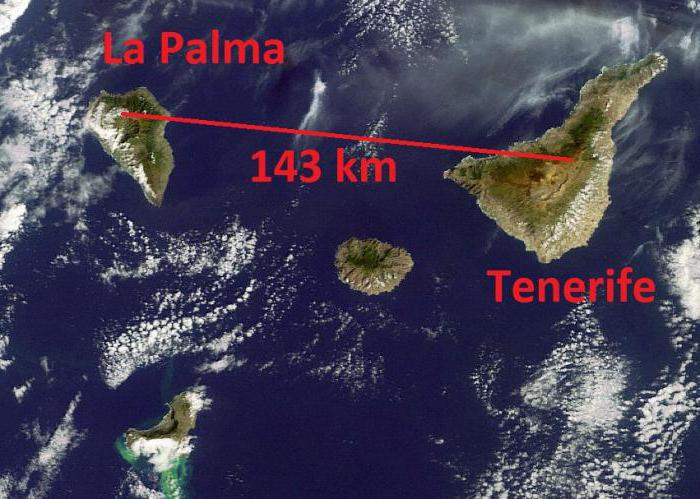Believe me, there is nothing difficult in solving problems. But provided that you know mathematics and have time for it. You will need knowledge of operations with fractions, the ability to count, open parentheses, and solve equations. Without such skills, physics will be very difficult.

## Real life examples

What is distance? As indicated by the distance in physics, we have already figured out. Now let's deal with the concept.

Imagine that you are now standing near your house. Your task is to get to school. The road is straight all the time. Walk on the strength for about two minutes. From the entrance doors to the school doors 200 meters. This is distance. What would the description of your walk from home to school look like?

S = 200 m.

Why didn't we write "meters", but limited ourselves to just a letter? Because such is the abbreviated letter designation. A little later, we will get acquainted with other parameters that are related to distance.Now imagine that the path from home to store is winding. If you look at the map of your area, you will see that the distance to the store from the house is the same as to the school. But why is the path so long? Because the road is not straight. You have to cross at a traffic light, go around a huge residential building, and only then you get to the store. In this case, the actual distance will be much greater. In geometry and physics, this means "crooked path". And a straight line is just pure distance, like walking through the wall of a large house. You can also give an example with a man who goes to work.

## What is the reason for the distance?

The concept of "distance" cannot exist on its own, it must play some role. For example, you are cycling to school instead of walking because you are late. As we said earlier, our path to school is straight. You can safely drive along the sidewalk. Naturally, walking will take longer than cycling. What is the matter here? This, of course, is about the speed with which you move. Later we will see formulas that will tell you how to find the distance. Physics is a science in which you have to calculate something. Agree, I wonder how fast you ride your bike? If you know the exact distance to school and the travel time, you will also find the speed.

So, we have two more parameters:

t - time,

v - speed.Everything will be much more interesting if you learn to work with formulas and find the unknown using fractions. Let us recall just a rule from mathematics: everything that is next to the unknown goes to the denominator (that is, down the fraction). For example, the formula for distance (physics) is the product of time and speed. In other cases, fractions. Look at the picture that shows how to find distance, speed and time. Be sure to practice and figure out how such formulas are obtained. Everything follows only from the laws of mathematics, there is nothing invented in these formulas. Let's practice (don't peep): what letter denotes distance in physics?

## How are they measured?

Let's hope that you remember the designation of the main quantities, their designations. It's time to study the units of measurement. Here, too, you will have to train your memory, memorize. It is important to know not only how distance is indicated in physics, but also time, speed. But this is just a small topic. It will be more difficult further. Let's get started:

S - distance - meter, kilometer [m], [km];

v - speed - meters per second, kilometers per hour [m / s], [km / h] (in the case of cosmic speeds, a kilometer per second can be used;

t - time - second, minute, hour [s], [min], [h].Pay attention to how speed is indicated. That's right, a fraction. Now imagine this: S / t = m / s or S / t = km / h. This is where the fractions come from. In the SI system of international units, these parameters have the following values: meter, second, meter per second.

We figured out how distance is denoted in physics, considered time and speed, which are inextricably linked with it.

In this lesson, we will look at three physical quantities, namely distance, speed and time.

## Distance

We have already studied distance in the unit of measurement lesson. In simple terms, distance is the length from one point to another. (Example: the distance from home to school is 2 kilometers).

When dealing with long distances, they will mainly be measured in meters and kilometers. Distance is indicated by a Latin letter S... You can also designate another letter, but the letter Sgenerally accepted.

## Speed

Speed ​​is the distance traveled by the body per unit of time. The unit of time means 1 hour, 1 minute, or 1 second.

Suppose that two schoolchildren decide to check who will run faster from the yard to the sports ground. The distance from the courtyard to the sports ground is 100 meters. The first student ran in 25 seconds. Second in 50 seconds. Who ran faster?

The one who ran the greater distance in 1 second ran faster. They say that he has a faster movement speed. In this case, the speed of schoolchildren is the distance they run in 1 second.

To find the speed, you need to divide the distance by the travel time. Let's find the speed of the first student. To do this, we divide 100 meters by the time of movement of the first student, that is, by 25 seconds:

100 m: 25 s = 4

If the distance is given in meters, and the time of movement is in seconds, then the speed is measured in meters per second. (m / s). If the distance is given in kilometers and the travel time is in hours, the speed is measured in kilometers per hour. (km / h).

Our distance is given in meters, and time is in seconds. This means the speed is measured in meters per second (m / s)

100m: 25s = 4 (m / s)

So, the speed of movement of the first student is 4 meters per second (m / s).

Now let's find the speed of movement of the second student. To do this, we divide the distance by the time of movement of the second student, that is, by 50 seconds:

100 m: 50 s = 2 (m / s)

This means that the speed of movement of the second student is 2 meters per second (m / s).

The speed of the first student - 4 (m / s) The speed of the second student - 2 (m / s)

4 (m / s)> 2 (m / s)

The speed of the first student is higher. So he ran to the sports ground faster. Speed ​​is indicated by a Latin letter v.

## Time

Sometimes a situation arises when it is required to find out how long it takes for a body to cover a particular distance.

For example, the distance from home to the sports section is 1000 meters. We have to get there by bike. Our speed will be 500 meters per minute (500m / min). How long will it take to get to the sports section?

If we drive 500 meters in one minute, then how many minutes with five hundred meters will there be in 1000 meters?

Obviously, we need to divide 1000 meters by the distance that we will travel in one minute, that is, 500 meters. Then we get the time it takes to get to the sports section:

1000: 500 = 2 (min)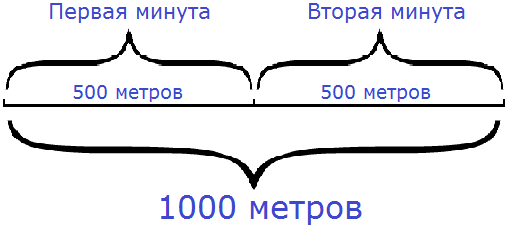The time of movement is indicated by a small Latin letter t.

## The relationship of speed, time, distance

Speed ​​is usually denoted by a small Latin letter v, movement time - in small letter tdistance traveled - in small letter s... Speed, time and distance are related.

If the speed and time of movement are known, then the distance can be found. It is equal to speed times time:

s = v × t

For example, we left the house and headed to the store. We reached the store in 10 minutes. Our speed was 50 meters per minute. Knowing our speed and time, we can find the distance.

If we walked 50 meters in one minute, then how many such fifty meters will we cover in 10 minutes? Obviously, multiplying 50 meters by 10, we will determine the distance from home to the store:

v = 50 (m / min)

t = 10 minutes

s = v × t = 50 × 10 = 500 (meters to the store)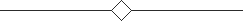If you know the time and distance, then you can find the speed:

v = s: t

For example, the distance from home to school is 900 meters. The schoolboy reached this school in 10 minutes. How fast was it?

The student's speed is the distance he travels in one minute. If he covered 900 meters in 10 minutes, what distance did he cover in one minute?

To answer this, you need to divide the distance by the time the student is moving:

s = 900 meters

t = 10 minutes

v = s: t = 900: 10 = 90 (m / min)If you know the speed and distance, then you can find the time:

t = s: v

For example, the distance from home to the sports section is 500 meters. We have to walk to her. Our speed will be 100 meters per minute (100 m / min). How long will it take to get to the sports section?

If we walk 100 meters in one minute, then how many minutes with one hundred meters will there be in 500 meters?

To answer this question, you need to divide 500 meters by the distance that we will cover in one minute, that is, by 100. Then we will get the time in which we will reach the sports section:

s = 500 meters

v = 100 (m / min)

t = s: v = 500: 100 = 5 (minutes before the sports section)